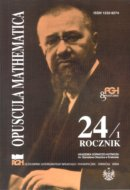Opuscula Math. 24, no. 1 (2004), 103-114

Opuscula Mathematica

# Li's criterion for the Riemann hypothesis - numerical approach

Krzysztof Maślanka

Abstract. There has been some interest in a criterion for the Riemann hypothesis proved recently by Xian-Jin Li [Li X.-J.: The Positivity of a Sequence of Numbers and the Riemann Hypothesis. J. Number Theory 65 (1997), 325-333]. The present paper reports on a numerical computation of the first 3300 of Li's coefficients which appear in this criterion. The main empirical observation is that these coefficients can be separated in two parts. One of these grows smoothly while the other is very small and oscillatory. This apparent smallness is quite unexpected. If it persisted till infinity then the Riemann hypothesis would be true.

Keywords: Riemann zeta function, Riemann hypothesis, Li's criterion, numerical methods in analytic number theory.

Mathematics Subject Classification: 11M26, 11Y60.

Full text (pdf)

• Krzysztof Maślanka
• Astronomical Observatory of Jagiellonian University, ul. Orla 171, 30-244 Cracow, Poland